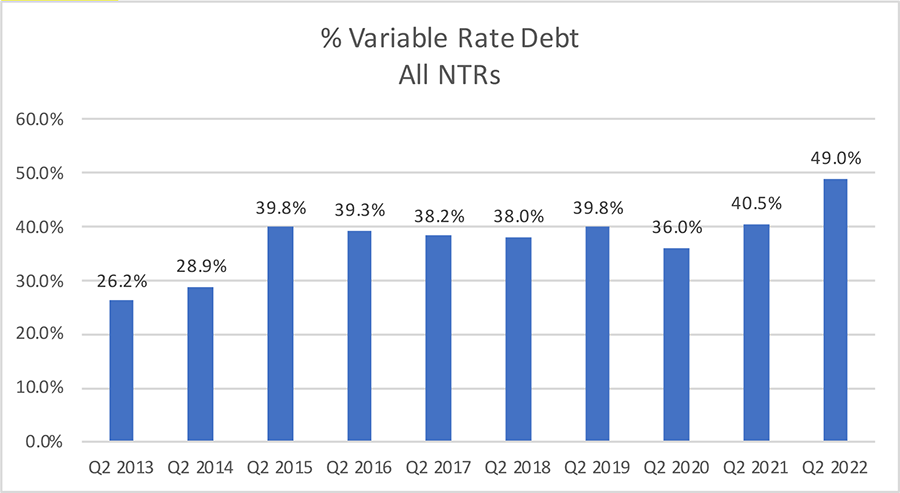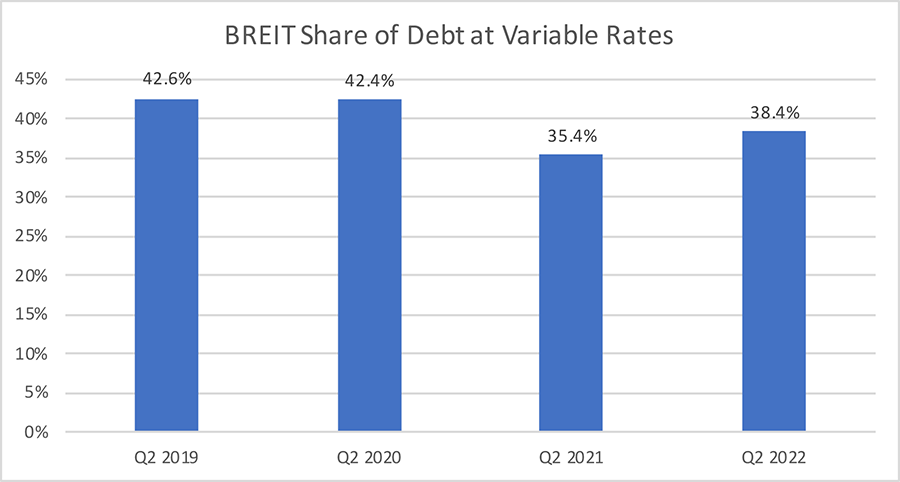October 25, 2022

# Interesting Trends in Nontraded REIT DebtOctober 25, 2022

# Interesting Trends in Nontraded REIT Debt

October 25, 2022 | James Sprow | Blue Vault

As expected with the economy-wide rise in interest rates, the weighted average interest rates on nontraded REIT (NTR) debt as reported in the Q2 2022 financials filed by NTRs have increased after reaching their quarterly low in Q1 2022.  The chart below shows the bottoming out of those weighted average interest rates for the Q1 2022 reports, and the bounce upward in Q2 2022. All of these rates have been calculated weighting the rates by the debt held by each REIT. By using the weighting by total debt, large REITs such as Blackstone REIT have their weighted average interest rate on outstanding debt given more weight in the industry averages. Blackstone REIT’s weighted average interest rate on all outstanding debt as of Q2 2022 was 3.70%, up from 3.10% in Q2 2021.  The chart compares weighted average interest rates on a Y-O-Y basis for the second quarters each year going back to 2013.

Chart IAlong with the trend over the last year that shows increasing weighted average interest rates, there is an interesting anomaly that may be difficult to explain. In the most recent quarter for which data is available across the NTR industry, the proportion of debt that NTRs have on their books at unhedged variable rates has increased significantly. The following chart shows that the proportion of total NTR debt that is at unhedged variable rates has increased from 40.4% for Q2 2021 to 49.0% for Q2 2022.

We would expect that REITs would be dealing with the increase in interest rates in the U.S. economy by locking in more fixed rate debt, other things equal. However, as we will explain, it is possible for REITs to use hedging contracts like interest rate swaps and caps to limit their exposure to rising rates.

Chart IIDefining Variable Rate Debt

The proportion of NTR debt at unhedged variable rates was fairly consistent for seven years until the most recent quarter. How do we explain this sudden increase in the weighted average proportion of variable rate debt?  First, we must define unhedged variable rate debt. Blue Vault reports the unhedged variable rate debt as debt that has variable rates despite the use of interest rate caps. Much of the variable rate debt that NTRs have on their books is hedged with interest rate caps, but Blue Vault considers that debt as unhedged when the interest rates can rise until they reach their caps. For example, if a line of credit is at variable rates which are currently at SOFR + 1.00% (SOFR as of 10/18/22 was 3.04%) which would translate to 4.04%, it would be treated as unhedged variable rate debt if the cap is at 4.50%. Until the cap is reached, the debt’s interest rate can rise and is still treated as variable.

The other common method of converting variable rate debt to fixed rate debt is the use of interest rate swaps. A swap contract is a legal contract with a counterparty such as a bank where the REIT and the bank agree to exchange the interest expense obligation on the variable rate borrowings of the REIT for a fixed interest rate. For example, if the REIT has that same line of credit at SOFR+1.00%, it can contract with a counterparty to swap the interest payments due on that debt for a fixed rate of 4.00%. If the rate on the variable rate line of credit goes up with SOFR to 5.00%, the counterparty is committed to paying that rate while the REIT’s interest payments are based upon the 4.00% swap rate. Swaps effectively convert variable rate debt to fixed rate debt and we do not report it as variable rate debt in our Blue Vault NTR reports.

The Impact of Blackstone REIT on NTR Debt Totals

We cannot ignore the impact of Blackstone Real Estate Income Trust (BREIT) on the NTR industry data. The chart below shows the share of all NTR assets owned by BREIT. That share has increased from 20.1% in Q2 2019 to 56.4% in the most recent reported quarter.

Chart IIIAlong with the significant increase in the share of NTR total assets on the BREIT books, the amount of variable rate debt carried by BREIT is also a large contributor to the industry’s proportion of variable rate debt.

Chart IVClearly, BREIT’s use of unhedged variable rate debt could increase the proportion for the entire NTR industry. However, BREIT has used \$22.4 billion in swap notional value to swap its variable rate debt for fixed debt. While the REIT’s variable rate debt is a significant portion of the NTR industry’s debt, that share does not appear to impact the industry’s ratio of variable rate debt to total debt.

What we must keep in mind is that, as interest rates continue to rise, more of the variable debt’s rates will reach the caps that the REITs, such as BREIT, have in place. As of June 30, 2022, BREIT had notional amounts of interest rate caps of \$14.8 billion, with a strike of 3.5%. As rates rise, these interest rate caps will become binding, meaning they will effectively fix the rates on a significant amount of the REIT’s total mortgage notes, term loans and secured revolving credit facilities, debt which totaled \$53.1 billion at June 30, 2022. When interest rate caps are binding, the proportion of the REIT’s debt at unhedged variable rates could drop significantly.

Sources: SEC, Blue Vault